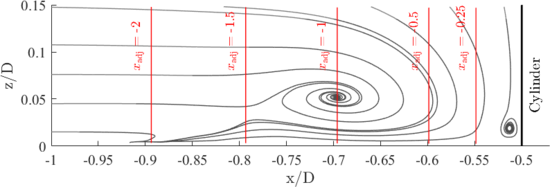# Evaluation

The evaluation of the numerical and experimental data sets in the symmetry plane upstream a wall-mounted cylinder refers to the time-averaged flow fields indicated by:

• the streamlines
• the location of the characteristic flow structures
• selected vertical and horizontal profiles of the velocity components $u(z)$and $w(x)$as well as the Reynolds sresses $u'_{i}u'_{j}$and the trubulent kinetic energy $k(z)$• the distribution of the turbulent kinetic energy and its buget terms such as production, transport, and dissipation
• horizontal profiles of the pressure coefficient $c_{\mathrm {p} }(x)$## Streamlines

The streamlines of the PIV and the LES data show high similarity and agree well with each other. The approaching turbulent boundary layer is redirected downwards at the flow facing edge of the cylinder caused by a vertical pressure gradient. This downflow reaches the bottom plate of the flume at the stagnation point S3. Here, it becomes redirected (i) in the out-of-plane direction bending around the cylinder; (ii) towards the cylinder rolling up and forming the corner vortex V3; and (iii) in the upstream direction accelerating and fomring a wall-parallel jet. Due to the strong acceleration of the jet, a high vertical velocity gradient is exerted onto the bottom plate, which induces high wall shear stress. Parts of the approaching boundary layer is dragged downwards forming the horseshoe vortex V1. Upstream of thsi vortex system, the approaching flow is blocked and recirculates, and as a consequence a saddle point S1 with zero velocity magnitude appears.

## Location of the characteristic flow structures

The position of the characteristic flow structures highlited in the streamline plots is listed in the following table

Position of flow structures
PIV LES
$x/D$$z/D$$x/D$$z/D$S1 $-0.788$$0.03$$-0.843$$0.037$S2 $-0.918$$0$$-1.1$$0$S3 $-0.533$$0$$-0.534$$0$S4 $-0.507$$0.036$$-0.50$$0.04$V1 $-0.697$$0.051$$-0.735$$0.06$V3 $-0.513$$0.017$$-0.513$$0.02$## Profiles of the velocity components and Reynolds stresses

The vertical profiles are extracted at the following positions with respect to the time-averaged position of the horseshoe vortex, and can be found in the following plot.Since the flow structure of the LES and the PIV is slightly different, we use an adjusted $x-$coordinate, in order to compare the data at the same position in the flow. The coordinate is defined as follows:

$x_{\mathrm {adj} }={\frac {x-x_{\mathrm {Cyl} }}{x_{\mathrm {Cyl} }-x_{\mathrm {V1} }}}$,

with $x_{\mathrm {Cyl} }=-0.5D$, such that $x_{\mathrm {adj} }=-1.0$represents the time-averaged location of the horseshoe vortex centre $x_{\mathrm {V1} }$.

## Horizontal profiles of the pressure coefficient $c_{\mathrm {p} }(x)$Contributed by: Ulrich Jenssen, Wolfgang Schanderl, Michael Manhart — Technical University Munich